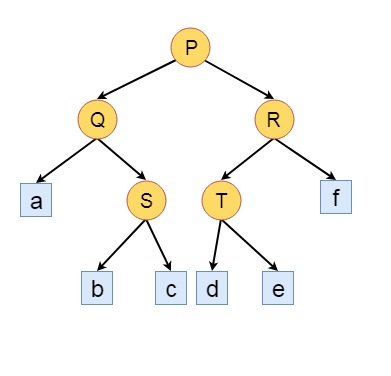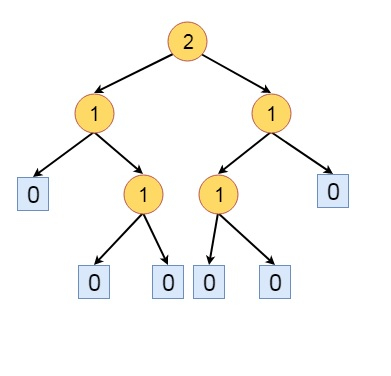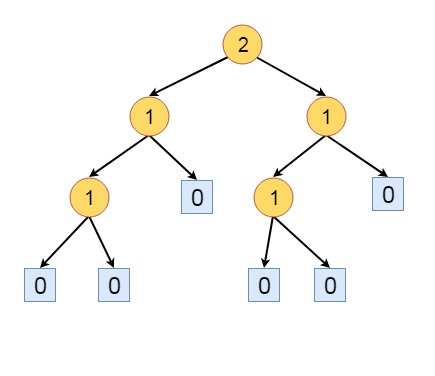# Height-Biased Leftist Trees in Data Structure

Here we will see what is the Height Balanced Leftist Trees (HBLT). Consider a binary tree where a special node, called an external node replaces each empty subtree. All other nodes are called Internal Nodes. When some external nodes are added with some binary tree, then that is called an extended binary tree.If we do not consider the leaf edges of this tree, then that is the actual binary tree. and this is the extended binary tree.

Now suppose s(x) be the length of a shortest path from node x to an external node in its subtree. If x is an external node, its s(x) value is 0. If the x is an internal node, the value is −

min{𝑠(𝐿), 𝑠(𝑅)} + 1

Here L and R are the left and right children of x. Now let us see the s values of the given tree.The definition of the HBLT is like: A binary tree is a Height Balanced Leftist Tree (HBLT), if and only if, at every internal node, the s value of the left child is greater or equal to the s value of right child.

The above tree is not HBLT. The parent of node a, has s(L) = 0, and s(R) is 1, except that all other nodes are satisfying the rule of the HBLT. So if we left and right subtree of that node, to make it HBLT.Some other definitions are −

• A max tree (min tree) is a tree, in which the value of each node is greater (less) or equal to its children.

• A max HBLT is an HBLT, that is also a max tree, a min HBLT is an HBLT, that is also a min tree.